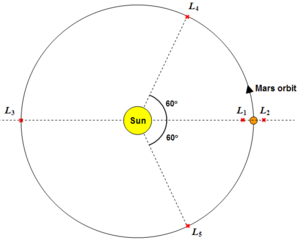# Lagrangian point

## Definition

A Lagrangian point is a point of interplanetary gravitational stability in a two body orbital configuration. Devised by the Italian/French mathematician and astronomer, Joseph Louis Lagrange (1736-1813), Lagrangian points are currently used to describe the influence of the planets over local space, provides a location for Trojan asteroids and may possibly be used by future space missions as a "stepping stone" to Mars and the Moon.

## Positions of the Lagrangian pointsExample: The position of the L1, L2, L3 and L4,5 points in the Mars-Sun system.

The simplest Lagrangian point to understand is the "first Lagrangian" (or $L_{1}$) point between the Earth and the Sun. The point at which the gravitational pull of the Sun and the gravitational pull of the Earth cancels out ($g_{Earth}+g_{Sun}=0$) creates an island of gravitational stability where space observatories, or indeed space stations, can be positioned.

$L_{2}$is located on the opposite side of the smallest orbital body (in this case, the Earth) to $L_{1}$. This very stable region is also useful to space observatories observing the cosmos. The Earth in this case will be constantly eclipsing the Sun, allowing sensitive optics to operate free of noise emitted from the Sun.

$L_{3}$is a less-stable Lagrangian point on the far side of the Sun. In this case, the Earth's gravitational force is negligible, allowing other planet's orbits to interfere with the gravitational stability of the region.

$L_{4}$and $L_{5}$are points leading and trailing the orbiting body at an angle of approximately 60° from the Earth-Sun line. These points are also known as "Trojan points" where asteroids (known as Trojan asteroids) become captured by the relative gravitational stability and orbit with the orbital body.

### Calculating the position of $L_{1}$and $L_{2}$If the mass of the larger body is massively greater than that of the orbiting body (as is the case in the Earth, or Mars, around the larger Sun), the following equation can be applied:

$r\approx R{\sqrt[{3}]{\frac {M_{2}}{3M_{1}}}}$where $r$is the distance of $L_{1}$or $L_{2}$from the orbiting body, $R$is the distance between the bodies and $M_{2}$and $M_{1}$are the masses of the smaller and larger bodies respectively.

## Current missions using the Lagrangian points of the Earth-Sun system

• $L_{1}$• $L_{2}$## Objects observed in the Mars-Sun $L_{4}$and $L_{5}$points

Asteroids in the $L_{4}$and $L_{5}$Mars-Sun Lagrangian points are often called Mars Trojan asteroids. A handful of asteroids are in stable solar orbits, leading ($L_{4}$) and following ($L_{5}$) the path of Mars including an asteroid named "1999 UJ7" (at $L_{4}$) and "5261 Eureka" (at $L_{5}$).

## Uses of the Mars $L_{1}$and $L_{2}$points

• An "early warning system" to notify settlers about the onset of solar storms.
• Communications satellites inserted in stable orbits around the Lagrangian points - long-term nodes of communication between Earth and Mars. Could be extended to include the $L_{4}$and $L_{5}$points to allow communication even when Mars is in conjunction.
• A manned space station acting as a "staging post" for missions to asteroids or refuge before making the final plunge into Mars' gravitational well.